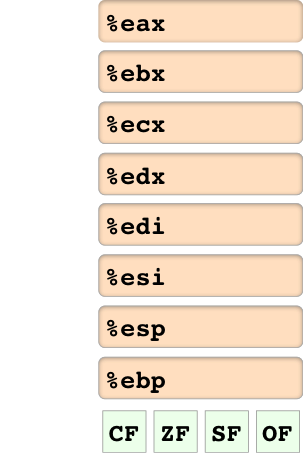hello

# Registers

int add(int x, int y) {

return x + y;
}


$\leftrightarrow$

add:
mov  %edi, %eax
retq


## Arithmetic Instructions

x86-64

add  src, dst
sub  src, dst
imul src, dst


$\leftrightarrow$

C Pseudocode

dst += src
dst -= src
dst *= src


src is register/immediate/memory

dst is register/memory# Division

x86-64

idivq denom

cqto


$\leftrightarrow$

C Pseudocode

%rax = (%rdx:%rax) / denom
%rdx = (%rdx:%rax) mod denom
%rdx:%rax = (int128_t)%rax


denom is a register

idiv simultaneously finds the remainder and the quotient

a:b means “concatenate the bits of a and b to get a single number”